# Step by Step guide for solving Rubik's Cube

Jan. 6, 2013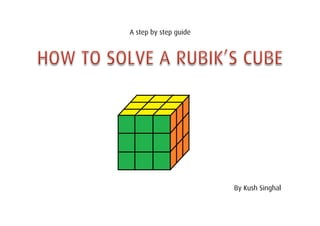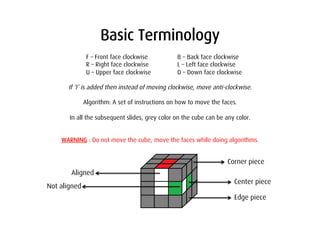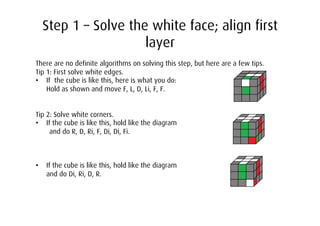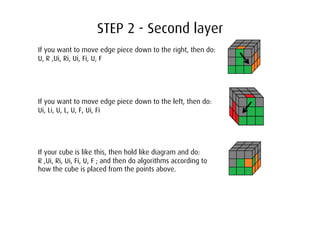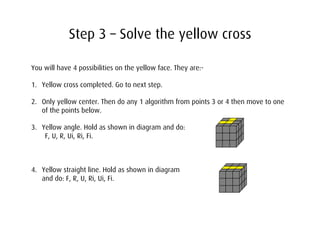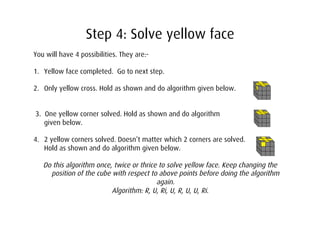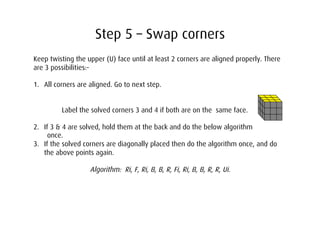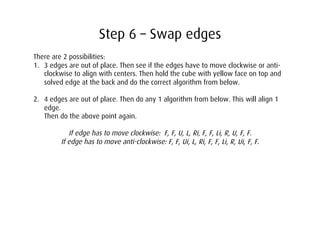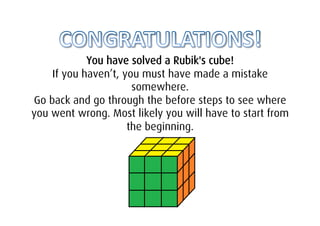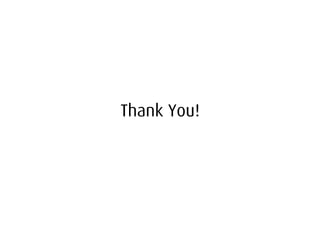1 of 10

### Step by Step guide for solving Rubik's Cube

• 1. A step by step guide By Kush Singhal
• 2. Basic Terminology F – Front face clockwise B – Back face clockwise R – Right face clockwise L – Left face clockwise U – Upper face clockwise D – Down face clockwise If ‘I’ is added then instead of moving clockwise, move anti-clockwise. Algorithm: A set of instructions on how to move the faces. In all the subsequent slides, grey color on the cube can be any color. WARNING : Do not move the cube, move the faces while doing algorithms. Corner piece Aligned Center piece Not aligned Edge piece
• 3. Step 1 – Solve the white face; align first layer There are no definite algorithms on solving this step, but here are a few tips. Tip 1: First solve white edges. •  If the cube is like this, here is what you do: Hold as shown and move F, L, D, Li, F, F. Tip 2: Solve white corners. •  If the cube is like this, hold like the diagram and do R, D, Ri, F, Di, Di, Fi. •  If the cube is like this, hold like the diagram and do Di, Ri, D, R.
• 4. STEP 2 - Second layer If you want to move edge piece down to the right, then do: U, R ,Ui, Ri, Ui, Fi, U, F If you want to move edge piece down to the left, then do: Ui, Li, U, L, U, F, Ui, Fi If your cube is like this, then hold like diagram and do: R ,Ui, Ri, Ui, Fi, U, F ; and then do algorithms according to how the cube is placed from the points above.
• 5. Step 3 – Solve the yellow cross You will have 4 possibilities on the yellow face. They are:- 1.  Yellow cross completed. Go to next step. 2.  Only yellow center. Then do any 1 algorithm from points 3 or 4 then move to one of the points below. 3.  Yellow angle. Hold as shown in diagram and do: F, U, R, Ui, Ri, Fi. 4.  Yellow straight line. Hold as shown in diagram and do: F, R, U, Ri, Ui, Fi.
• 6. Step 4: Solve yellow face You will have 4 possibilities. They are:- 1.  Yellow face completed. Go to next step. 2.  Only yellow cross. Hold as shown and do algorithm given below. 3. One yellow corner solved. Hold as shown and do algorithm given below. 4.  2 yellow corners solved. Doesn’t matter which 2 corners are solved. Hold as shown and do algorithm given below. Do this algorithm once, twice or thrice to solve yellow face. Keep changing the position of the cube with respect to above points before doing the algorithm again. Algorithm: R, U, Ri, U, R, U, U, Ri.
• 7. Step 5 – Swap corners Keep twisting the upper (U) face until at least 2 corners are aligned properly. There are 3 possibilities:- 1.  All corners are aligned. Go to next step. Label the solved corners 3 and 4 if both are on the same face. 2.  If 3 & 4 are solved, hold them at the back and do the below algorithm once. 3.  If the solved corners are diagonally placed then do the algorithm once, and do the above points again. Algorithm: Ri, F, Ri, B, B, R, Fi, Ri, B, B, R, R, Ui.
• 8. Step 6 – Swap edges There are 2 possibilities: 1.  3 edges are out of place. Then see if the edges have to move clockwise or anti- clockwise to align with centers. Then hold the cube with yellow face on top and solved edge at the back and do the correct algorithm from below. 2.  4 edges are out of place. Then do any 1 algorithm from below. This will align 1 edge. Then do the above point again. If edge has to move clockwise: F, F, U, L, Ri, F, F, Li, R, U, F, F. If edge has to move anti-clockwise: F, F, Ui, L, Ri, F, F, Li, R, Ui, F, F.
• 9. You have solved a Rubik's cube! If you haven’t, you must have made a mistake somewhere. Go back and go through the before steps to see where you went wrong. Most likely you will have to start from the beginning.